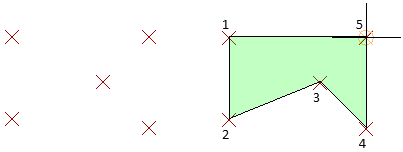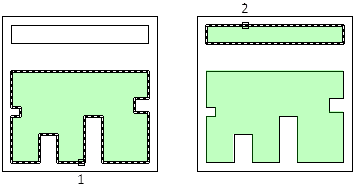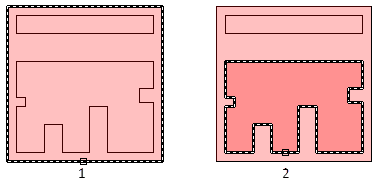# MEASUREGEOM (Command)

Measures the distance, radius, angle, area, and volume of selected objects or sequence of points.

Find

The MEASUREGEOM command performs many of the same calculations as the following commands:

• AREA
• DIST
• MASSPROP

Information displays at the Command prompt and in the dynamic tooltip in the current units format.

The following prompts are displayed.

### Distance

Measures the distance between specified points along with the X, Y, and Z component distances and the angle relative to the UCS.

Multiple points

Displays a running total of the distance between successive points.

If you enter the Arc, Length, or Undo options, additional options similar to those for creating a polyline are displayed.

Measures the radius and diameter of a specified arc, circle, or polyline arc.

### Angle

Measures the angle associated with selected arc, circle, polyline segments, and line objects.

Arc

Measures the angle formed between the two endpoints of an arc using the center of the arc as the vertex.

Circle

Measures the acute angle formed between where the circle was originally selected and a second point, using the center of the circle as the vertex.

Line

Measures the acute angle between two selected lines. The lines do not need to intersect.

Vertex

Measures the acute angle formed by specifying a point to act as a vertex and two other points.

### Area

Measures the area and perimeter of an object or defined area.

Specify corner points

Calculates the area and perimeter defined by specified points.If you enter the Arc, Length, or Undo options, additional options similar to those for creating a polyline are displayed.

Turns on Add mode and keeps a running total of area as you define areas.Subtract area

Subtracts a specified area from the total area.### Volume

Measures the volume of an object or a defined area.

Object

Measures the volume of an object or defined area.

You can select a 3D solids or 2D objects. If you select a 2D object you must specify a height for that object.

If you define an object by specifying points, you must specify at least three points to define a polygon. All must lie on a plane parallel to the XY plane of the UCS. If you do not close the polygon, an area will be calculated as if a line existed between the first and last points entered.

If you enter the Arc, Length, or Undo options, additional options similar to those for creating a polyline are displayed.

Turns on Add mode and keeps a running total of the volume as you define areas.

Subtract volume

Turns on Subtract mode and subtracts a specified volume from the total volume.

### Polyline Options

You can specify options similar to those used in creating polylines when you use the Distance, Area, and Volume options.

Arc

Adds arc segments to the total length.

Endpoint of arc

Completes the arc segment, which is tangent to the previous segment.

Angle

Specifies the included angle of the arc segment from the start point.

Entering a positive number creates counterclockwise arc segments. Entering a negative number creates clockwise arc segments.

• Endpoint of arc

Specifies the radius of the arc segment.

Center

Specifies the center of the arc segment.

• Endpoint of arc
• Angle
Length

Specifies the chord length of the arc segment. If the previous segment is an arc, the new arc segment is drawn tangent to the previous arc segment.

Close

Draws an arc segment from the last point specified to the starting point, creating a closed polyline. At least two points must be specified to use this option.

Direction

Specifies a starting direction for the arc segment.

• Endpoint of arc

Specifies the radius of the arc segment.

• Endpoint of arc
• Angle
Second pt

Specifies the second point and endpoint of a three-point arc.

• Endpoint of arc
Length

Draws a line segment of a specified length at the same angle as the previous segment. If the previous segment is an arc, the new line segment is drawn tangent to that arc segment.

Undo

Removes the most recent arc segment added to the polyline.

Close

Draws an arc segment from the last point specified to the starting point, creating a closed polyline. At least two points must be specified to use this option.